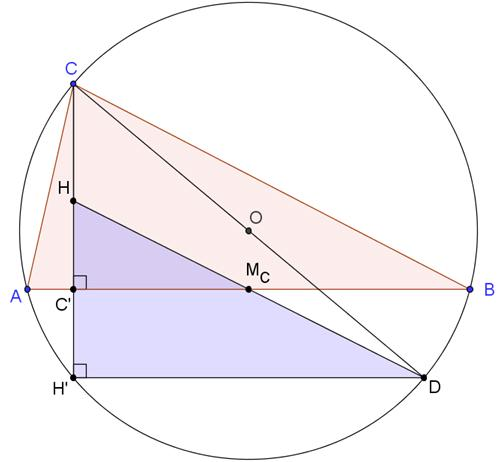# Reflections of the Orthocenter II

### Vladimir Nikolin an elementary school teacher from Serbia April 23, 2010

Reflections of the orthocenter in the sidelines of a triangle lie on the circumcircle of the triangle.

Let H be the orthocenter in ΔABC. Let H' be the refection of H using line AB, and point D is the second end of the circumdiameter CD. Mc the midpoint of AB.Since CD is a diameter, ∠CBD = 90° (as an inscribed angle subtended by a diameter), implying BD ⊥ BC. Also, AH being an altitude in ΔABC, is perpendicular to BC: AH ⊥ BC. Therefore, AH||BD.

Similarly, BH is an altitude (BH ⊥ AC) while ∠CAD = 90°. Therefore AD||BH.

The quadrilateral ADBH is a parallelogram. The diagonals AB and DH cross at their common midpoint which means that the midpoint Mc of AB lies on DH and, in addition, HMc = McD.

Letting C' be the foot of the altitude CH, we have, by the construction, HC' = C'H'. In triangle DHH' C'Mc is the midline parallel to DH': C'Mc||DH'. And, since ∠BC'C = 90°, so too ∠DH'H = 90°. ∠DH'C (= ∠DH'H) is right and subtended by the circumdiameter CD so, by Thales' theorem H' lies on the circumcircle, as required.

Note: a different proof appears elsewhere.### The Orthocenter